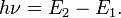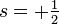# Atom

An atom (from the Greek atomos, indivisible) is the smallest physical entity that can retain its chemical properties. As such, atoms are the building blocks of all the matter that we come in contact with on a daily basis. Individual atoms are so small (on the order of one ångstrom = 1⋅10−10 m) that it takes literally sextillions (1021) of them to form a three-dimensional object that we can see with the naked eye. In fact, atoms were once thought to be the fundamental building blocks of the entire universe. We now understand that atoms are made up of smaller subatomic-particles. Indeed, in the beginning of the 20th century it was found that an atom consists of a heavy positively charged "pit", christened the nucleus of the atom by Ernest Rutherford, and a number of negatively much lighter particle, called electrons. The negative charges of the electrons cancel the positive charge of the nucleus, so that an atom is an electrically neutral particle.

##  Introduction

 It would be a poor thing to be an atom in a universe without physicists, and physicists are made up of atoms. A physicist is an atom's way of knowing about atoms. —George Wald (1906-1997), Nobel Laureate in Physiology/Medicine, 1967

The idea of an atom dates back to the ancient Greeks who postulated that all matter in the universe was made of tiny indivisible chunks. While this concept had no empirical support at the time, the search for the most fundamental "chunks" in the universe has remained one of most important the driving forces in science to this day. The particles that today bear the name "atom" were first encountered by early chemists who discovered that they could not be broken down nor transformed through any chemical reaction.

The modern atom serves as the fundamental particle for chemistry and as the smallest stable structure for engineering. Nuclear energy is generated either by breaking an atom into two or more smaller atoms (fission), or by combining two smaller atoms into a larger one (fusion).

##  History of the Atom

### The nineteenth century

In the beginning of the nineteenth century men as John Dalton, Joseph-Louis Gay-Lussac and Amedeo Avogadro hypothesized that matter consists of minute particles, atoms as Dalton liked to call them, or molecules (a term preferred by Avogadro and already used by Lavoisier). However, the difference between the two concepts was not clear. One of the main topics of the first international chemistry conference, the historic 1860 Karlsruhe conference, was to clear up the confusion about the difference between atoms and molecules. Stanislao Cannizaro offered for the first time in the history of science, a very clear definition of atoms as distinguished from molecules. To him the atom was the smallest quantity of each element which enters as a whole into the molecules which contain it. His statements represented a most remarkable contribution to the clarification of the issues debated at the time concerning the relations between atoms and molecules in both organic and inorganic compounds.

###  Albert Einstein gives molecules a reality in his explanation of Brownian motion

Employing the kinetic theory of heat and a mathematical approach to changes in velocity characterized by random motion, in 1905 Albert Einstein gave a convincing account of the microscopically observable random motion of pollen particles suspended in a liquid, previously noted by the botanist Robert Brown, attributing the motion to random collisions of the pollen particles with the molecules of the liquid in thermal motion. 

###  J.J. Thomson's "Plum Pudding" Model

The first widely accepted model of the atom was J.J Thomson's Model of a positively charged cloud with negatively charged "corpuscles," electrons, interspersed throughout. Thomson proposed and searched for configurations for which these particles had normal modes of vibration and were stable. He built his model using only electrostatic forces, which required that the electrons be in constant motion, this led to further difficulties and he was never able to create a model that matched observed data.

###  Rutherford Model

Ernest Rutherford, one of Thomson's students, disproved his theory in his now famous scattering experiment by showing that there was a dense, positively charged core at the center of each atom. Rutherford postulated that the structure of an atom more closely resembled a solar system with the nucleus at the center and electrons orbiting around it. Unfortunately, the electromagnetic theory of James Clerk Maxwell formulated 50 years earlier, predicts that these orbits would be unstable, they would decay very quickly, while emitting a continuous spectrum of light. However, it was already known that each element emitted a unique spectrum of discrete lines. Rutherford could not explain why these orbits did not decay nor why atoms did not emit the continuous spectrum that these decays would have caused.

###  Bohr's model of the Hydrogen Atom

In 1913 Niels Bohr devised the first quantum mechanical model of a (one-electron) atom. He resolved the difficulties of Rutherford's model by making the non-classical assumption that there were only discrete and stationary energy states allowed for the electron. Further, Bohr conjectured that the angular momentum of the electron was constrained to be an integer times Planck's constant h divided by 2π. Since in Bohr's model an electron can only make jumps between the stationary states, the atomic spectral lines were explained by the discreteness of the energy differences of the states. Emission of a photon occurs when an electron falls down from a state with energy E2 to a state with lower energy E1. The frequency ν of the emitted photon is given by$h\nu = E_2 - E_1 .\,$

Absorption of a photon of energy hν occurs when hν "matches" an atomic energy difference. An electron in certain state jumps to a state of higher energy such that the energy difference is equal to hν. Clearly, this requirement puts a strict constraint on the frequencies of the photons that may be absorbed by the atom.

Bohr's theory was for one-electron atoms only, but he conjectured that many-electron atoms would have a similar structure. The extension of his theory to many-electron systems led a decade later to Heisenberg's matrix mechanics and Schrödinger's wave mechanics.

###  Today's view

For most popular purposes Bohr's model is an acceptable approximation. However, the quantum mechanical interpretation is that the electrons are spread out in various probability distributions (wave functions squared) around the nucleus, rather than actually orbiting it. The theories of chemical bonding, atomic spectroscopy, atomic scattering etc., require the quantum mechanical description. The Bohr model cannot begin to explain the phenomena observed in these fields.

##  Structure of the Atom

Atoms are made of a dense nucleus formed by combinations of the two nucleons (positively charged protons and zero charge neutrons) and surrounded by a much larger "cloud" of electrons. The vast majority of the volume of an atom is empty space. The number of protons contained in the nucleus determines the atomic number and in turn which element it is classified as. The number of neutrons further specifies the isotope number of that element. The number of electrons surrounding the nucleus is typically assumed to be equal to the number of protons in order to keep the entire atom electrically neutral. Atoms that are not neutral are called ions, they are designated by their charge in units of elementary charge, which is equal to the negative of the number of surplus electrons present around the atom. Thus an atom with one extra electron is charged -1, and one missing is charged +1.

###  Forces in the atom

The nucleus of the atom contains a number of positively charged particles (protons) with no counter balancing negatively charged particles that keep the nucleus stable. In order to explain the stability of the nucleus, scientists introduced two new elementary forces, next to the gravitational force and the electromagnetic force: the strong force and the weak force. We now understand that the nucleus is held together by the strong force despite the positive charges repelling each other by Coulomb's law. The negatively charged electrons which surround the atom are attracted to the positive nucleus by Coulomb's law.

###  Electron Quantum States

Electrons surround the nucleus of a stable atom, where to a good approximation each may be assuemed to be in a unique quantum mechanical state. These states of well defined energy are called atomic orbitals and are organized from the lowest energy to highest. Each orbit can be uniquely defined by four quantum numbers, n the principal quantum number, l the angular momentum quantum number, m the magnetic quantum number, and s the spin quantum number. Principal quantum numbers can only be positive integers; l is zero or a positive integer. Each shell has n² spatial orbitals which are grouped by angular momentum l into subshells. These subshells are often given the names 's', 'p', 'f', 'g', and 'h' rather than numerals. Each of these subshells contains (2l+1) orbitals with magnetic quantum number m which runs from −l,...,0,...l. Finally, each orbital can contain two electrons of different spin$s=+\tfrac{1}{2}$ and$s=-\tfrac{1}{2}$. For a total of 2n² electrons per shell.

The valence, or the highest energy shell which contains electrons, is responsible for most of an elements chemical properties.

An atom with electrons in higher energy states without first filling lower states is considered "excited". It can reach that state by absorbing one or more photons. An excited atom is likely to emit its excess energy in the form of a photon of energy equal to the difference between the current state and a lower energy state until it is no longer excited. These emissions are responsible for the emission and that atoms produce. In fact it is this property that allows us to discern the chemical composition of stars and planets from a great distance.

###  Nuclei Quantum States

The nucleus of an atom itself also has a shell-like structure not dissimilar to electron shells. This leads to some extra stable and semi-stable states when the number of protons or neutrons in the nucleus is equal to the "magic numbers" 20, 28, 50, 82, and 126 which is believed to correspond to completely filled shells.  Stable nuclei fall upon a "line" of stability which starts very close to equal numbers of protons and neutrons and move towards favoring neutrons for heavier nuclei. This is a result of the fact that the electrostatic repulsion of two protons drops in magnitude much more slowly than the residual strong force. Either way nucleons strongly prefer to bond in even numbers.

###  Decay

Most combinations of nucleons are inherently unstable and undergo a number of radioactive decays in order to form more stable nuclei. In all of the common decays a particle is emitted from the nucleus in order to compensate for some instability in the atom.

• Alpha decay most frequently occurs in atoms which are simply too big, atoms are limited in size because the residual strong force which holds them together only acts over very small distances so that the rate of electrostatic repulsion grows faster than the rate of strong attraction as the nucleus grows. Alpha decay emits an Alpha particle which is denoted with the Greek letter α.
• Beta+ decay occurs in atoms which are proton heavy. In this decay a proton decays into a neutron and emits both a positron and a neutrino. The only natural Beta+ emitter is 40K.
• Beta- decay occurs in atoms which are neutron heavy. In this decay a neutron decays into a proton and emits both an electron and a neutrino. A lone proton can beta- decay.
• Gamma decay occurs in atoms where the nucleus is in an excited state. The nucleus will turn this excess energy into a high energy photon, typically called a gamma ray. Gamma decays typically follow either an alpha or beta decay which leaves the nucleus with excess energy.
• Electron capture occurs when a proton inside the nucleus captures an electron and becomes a neutron.